# Generalized Gamma Distribution Example

This example appears in the Life Data Analysis Reference book.

The following data set represents revolutions-to-failure (in millions) for 23 ball bearings in a fatigue test, as discussed in Lawless .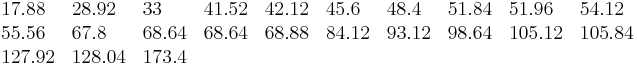When the generalized gamma distribution is fitted to this data using MLE, the following values for parameters are obtained: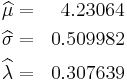Note that for this data, the generalized gamma offers a compromise between the Weibull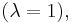and the lognormal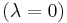distributions. The value of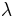indicates that the lognormal distribution is better supported by the data. A better assessment, however, can be made by looking at the confidence bounds on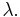For example, the 90% two-sided confidence bounds are:We can then conclude that both distributions (i.e., Weibull and lognormal) are well supported by the data, with the lognormal being the better supported of the two. In Weibull++ the generalized gamma probability is plotted on a gamma probability paper, as shown next.

It is also important to note that, as in the case of the mixed Weibull distribution, the choice of regression analysis (i.e., RRX or RRY) is of no consequence in the generalized gamma model because it uses non-linear regression.